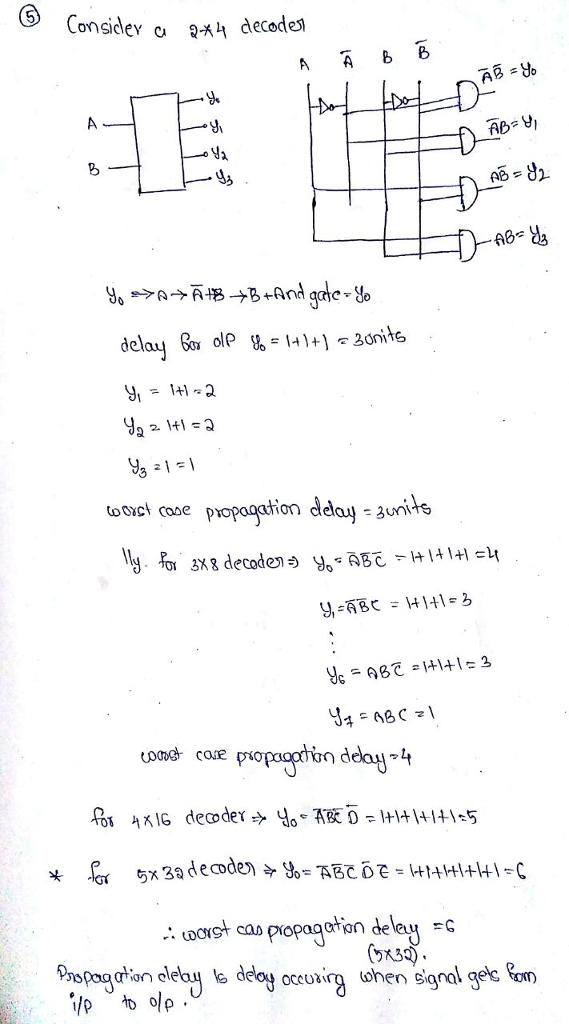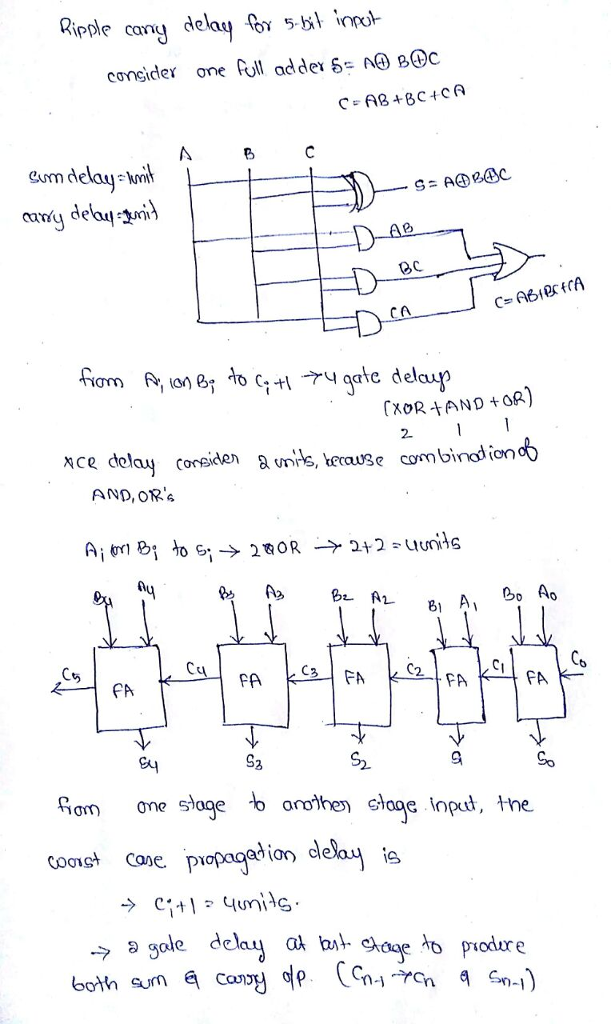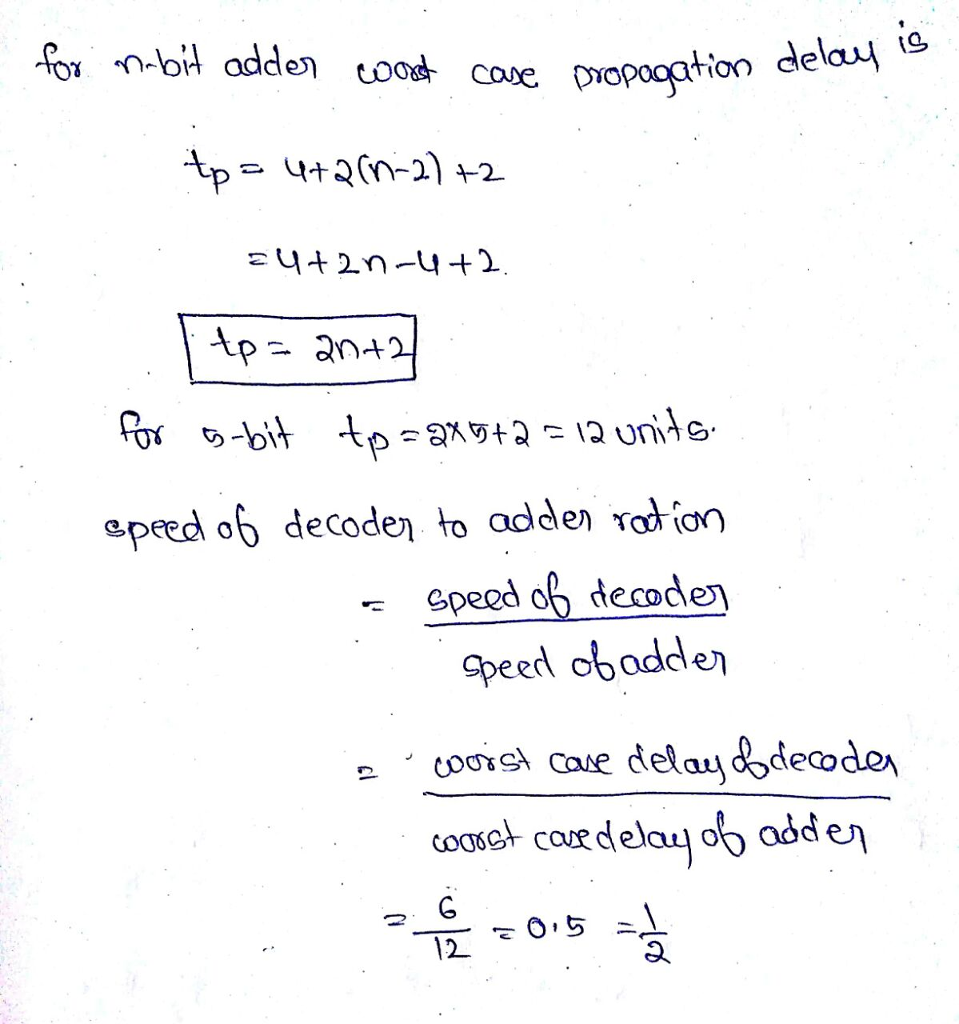# Homework Solution: What is the minimum number of gates required to construct a 5 times 32 decoder? What is the worst case propagateion delay for the decoder i…What is the minimum number of gates required to construct a 5 times 32 decoder? What is the worst case propagateion delay for the decoder in units of gate delays? How does this compare to the number of gates needed to build a 5-bit ripple-carry adder, an adder that accepts inputs represented using 5 bits, and to the worst case propagation delay adder? What is the ratio of speed decoder to adder?